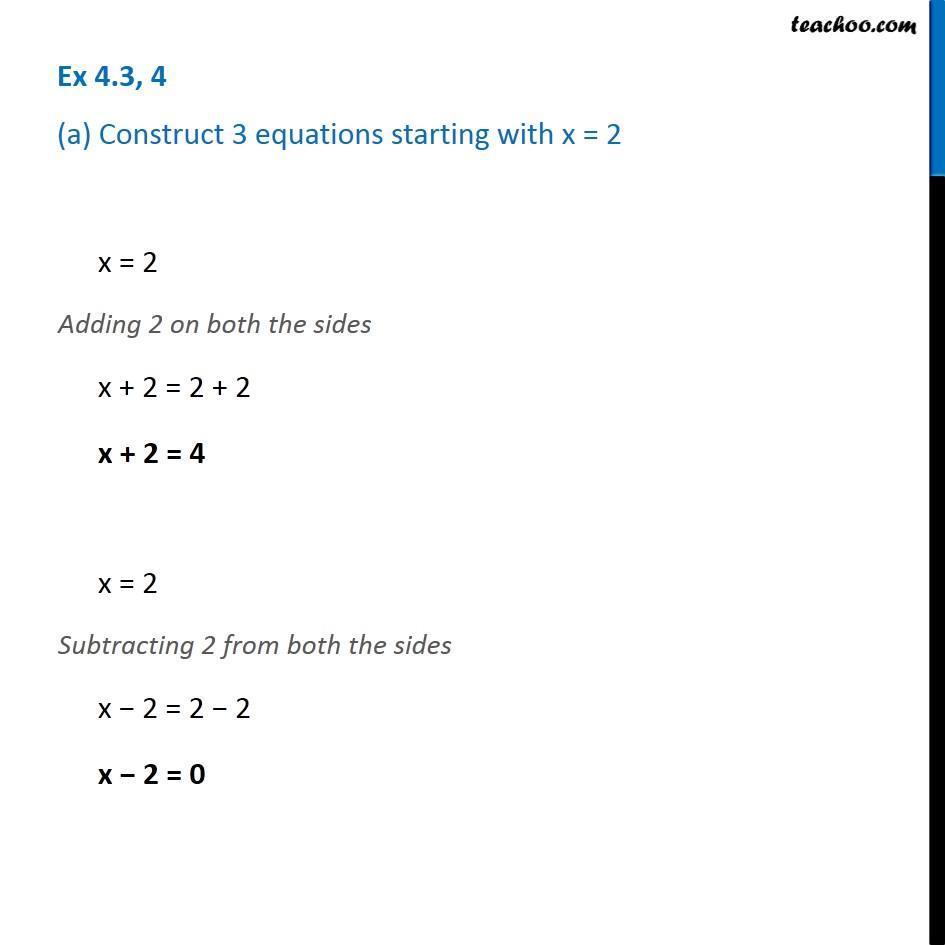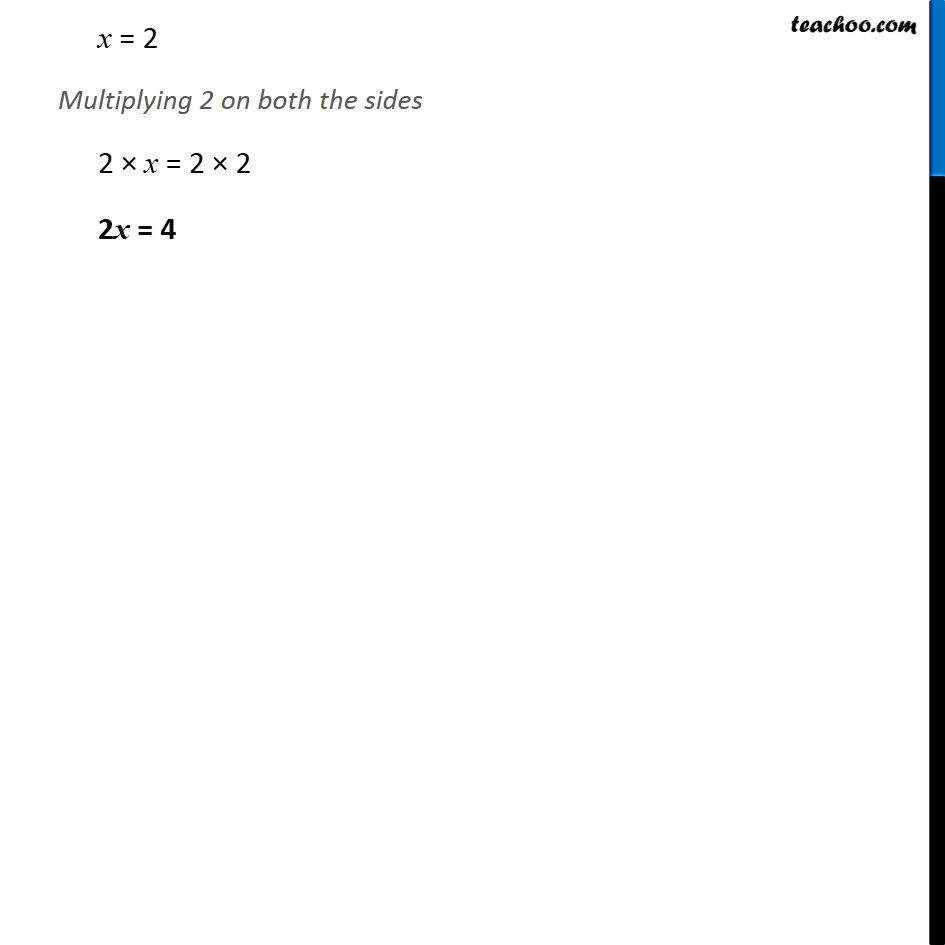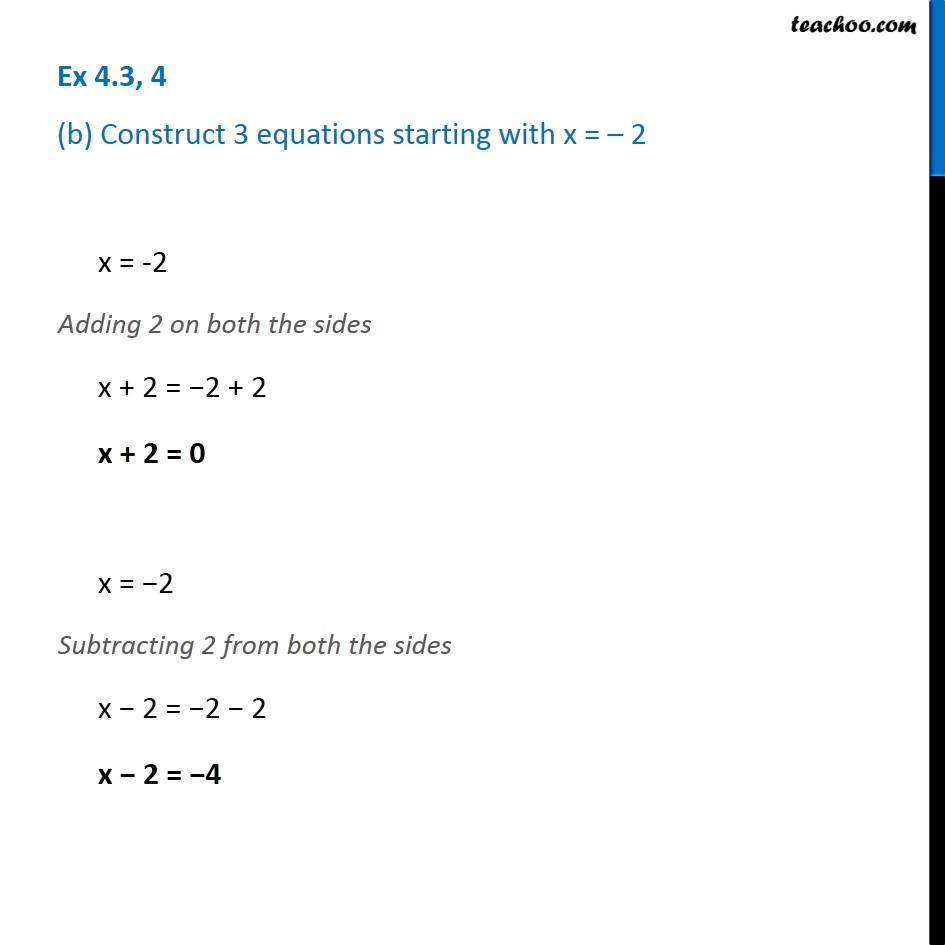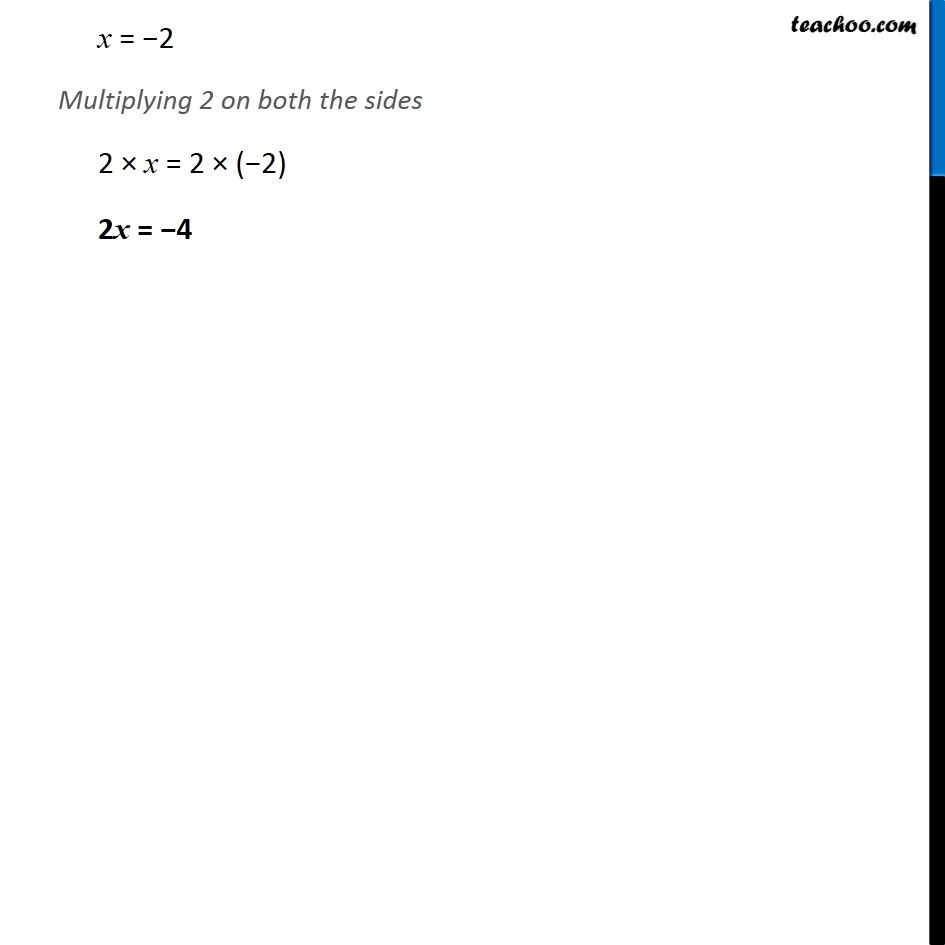1. Chapter 4 Class 7 Simple Equations
2. Concept wise
3. Solving Equations

Transcript

Ex 4.3, 4 (a) Construct 3 equations starting with x = 2x = 2 Adding 2 on both the sides x + 2 = 2 + 2 x + 2 = 4 x = 2 Subtracting 2 from both the sides x − 2 = 2 − 2 x − 2 = 0 x = 2 Multiplying 2 on both the sides 2 × x = 2 × 2 2x = 4 Ex 4.3, 4 (b) Construct 3 equations starting with x = – 2x = -2 Adding 2 on both the sides x + 2 = −2 + 2 x + 2 = 0 x = −2 Subtracting 2 from both the sides x − 2 = −2 − 2 x − 2 = −4 x = −2 Multiplying 2 on both the sides 2 × x = 2 × (−2) 2x = −4

Solving Equations